# How to Multiply a Fraction by a Whole Number

How to Multiply a Fraction by a Whole NumberTo multiply a fraction by a whole number, multiply the numerator on top of the fraction by the whole number but leave the denominator on the bottom the same.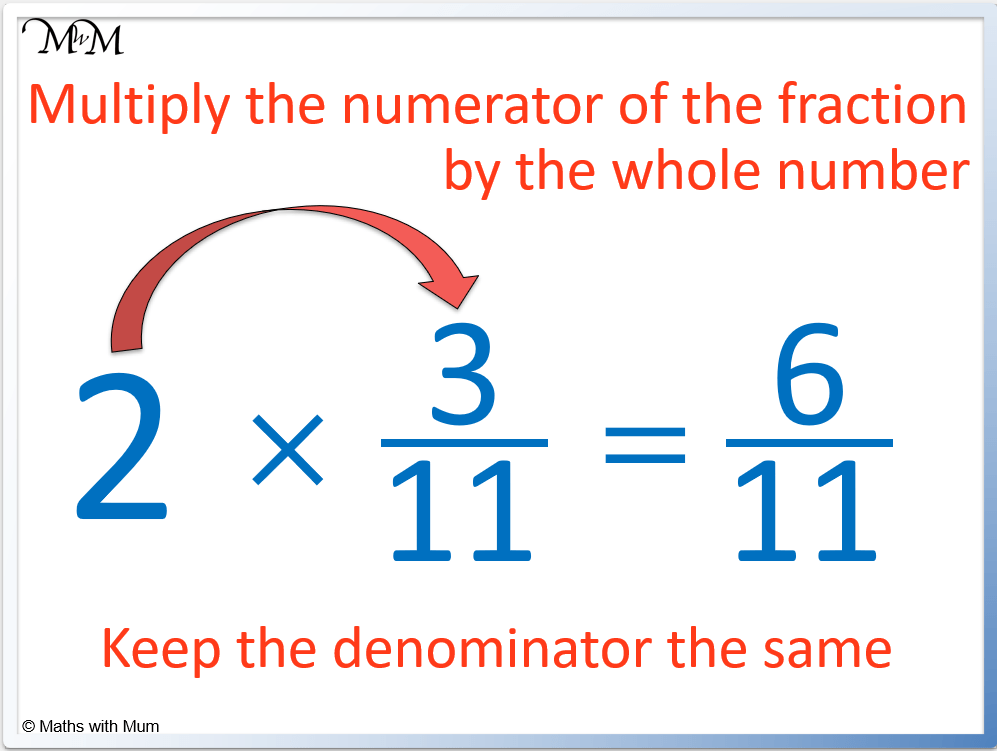• The numerator of the fraction is the number on the top, which is 3.
• Multiply the numerator of the fraction but not the denominator.
• 2 × 3 = 6 and so, the new numerator is 6.
• The denominator of 11 remains as 11.
• 2 ×   3/11   =   6/11  .

Alternatively, whole numbers can be written as a fraction out of 1.

Then multiply the numerators and denominators separately.• Another way to multiply a fraction by a whole number is to turn the whole number into a fraction.
• The whole number is written as a fraction out of 1.
• For example, 4 can be written as   4/1.
• The second step is to multiply the numerators on the top of the fractions and to multiply the denominators on the bottom of the fraction.
• 4 × 1 = 4 and 1 × 2 = 2 so   4/1   ×   1/2   =   4/2  .
• 4/2     can be simplified to   2/1  , which is the same as 2.• To multiply a fraction by a whole number, multiply the numerator by the whole number and leave the denominator the same.
• To multiply 5 ×   2/7   is found by multiplying the 2 by 5.
• 5 × 2 = 10 and so, 5 ×   2/7   =   10/7.Supporting Lessons# Multiplying Fractions and Whole Numbers

## How to Multiply a Fraction by a Whole Number

To multiply a fraction by a whole number:

1. Multiply the numerator of the fraction by the whole number.
2. Keep the denominator the same.
3. Simplify the fraction if possible.

For example, multiply 5 ×   2/7  .The numerator of the fraction is the number on top, which is 2.

We multiply 2 by 5 but keep the denominator of 7 as 7.

5 ×   2/7   =   10/7.

We simplify if possible. Since the fraction is an improper fraction, we can convert it into a mixed number.

10/7   means 10 ÷ 7, which is 1 remainder 3. Therefore the fraction can be written as 1   3/7.

## Alternative Method for Multiplying a Fraction by a Whole Number

To multiply a fraction by a whole number:

1. Write the whole number as a fraction out of 1.
2. Multiply the numerators.
3. Multiply the denominators..
4. Simplify if possible.

For example, multiply 4 ×   1/2.The first step is to write 4 as   4/1.

The second step is to multiply the numerators: 4 × 1 = 4. The numerator of the answer is 4.

The third step is to multiply the denominators: 1 × 2 = 2. The denominator of the answer is 2.

Therefore 4 ×   1/2   =   4/2.

Finally, simplify the fraction by dividing both the numerator and denominator by the same value. We can divide 4 and 2 by 2 so   4/2   simplifies to   2/1.   2/1   is the same as 2.

Therefore 4 ×   1/2   = 2.

We also know that one half of 4 is 2.

## How to Multiply a Fraction by a Whole Number in Simplest Form

To multiply a fraction by a whole number, multiply the numerator by the whole number. To write this answer in the simplest form, divide the numerator and denominator by the largest number that divides into both exactly.

For example, work out 2 ×   3/10   in simplest form.The first step is to multiply the numerator of the fraction by the whole number. 2 × 3 = 6 and so 2 ×   3/10   =   6/10.

The second step is to simplify the fraction by dividing the numerator and denominator by the largest number that divides into both.

Both 6 and 10 can be divided by 2.   6/10   simplifies to   3/5.

Therefore 2 ×   3/10   in simplest form is   3/5.

Multiplying a fraction by a whole number can also be calculated by dividing the denominator by the whole number.

10 is the denominator and 10 ÷ 2 = 5, which is the new denominator. This only works if the denominator of the fraction can be divided by the whole number.

## How to Multiply a Mixed Number by a Whole Number

To multiply a mixed number by a whole number:

1. Convert the mixed number into an improper fraction.
2. Multiply the numerator of the improper fraction by the whole number.
3. Simplify if possible and convert back to a mixed number.

For example, multiply 2 × 1   2/3.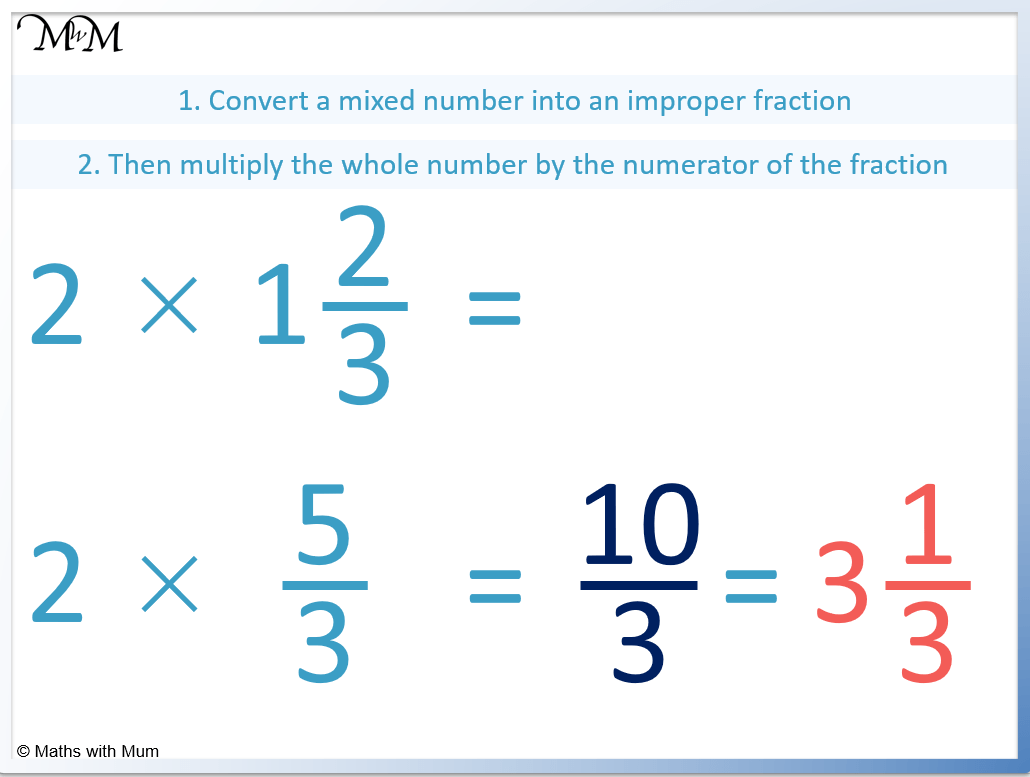Step 1. Convert the mixed number into an improper fraction.

Keep the denominator the same.

To find the new numerator, multiply the whole number of the mixed number with the denominator and then add the numerator.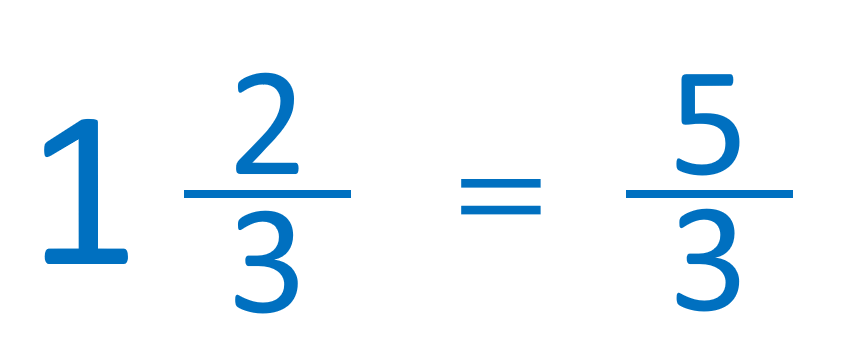The denominator is 3. The numerator is found by multiplying 1 and 3 to make 3 and then adding 2 to make 5.

1   2/3   =   5/3.

Step 2. Multiply the numerator of the improper fraction by the whole number.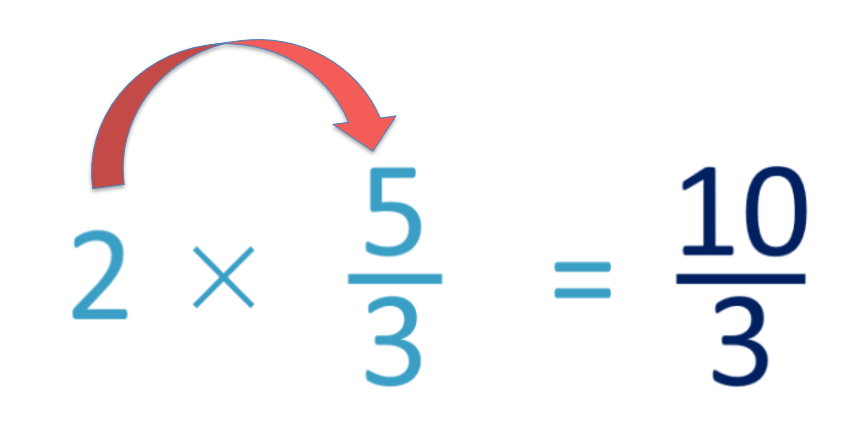We multiply 2 × 5 = 10 and so 2 ×   5/3   =   10/3.

The final step is to simplify and write as a mixed number again.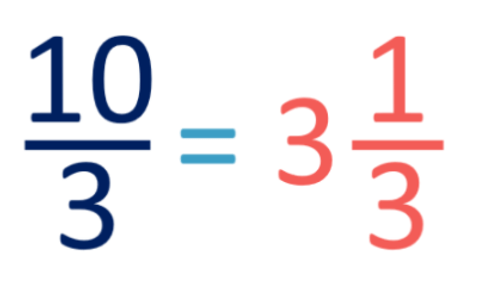10/3   = 3   1/3  .

Therefore 2 × 1   2/3   = 3   1/3

## Multiplying a Fraction by a Whole Number Using a Number Line

Mark the fraction on the number line. To multiply it by a whole number, add on the same fraction as many times as the multiplication requires.

For example here is 5 ×   1/8   on a number line.

We split each whole number into eighths and count five of them.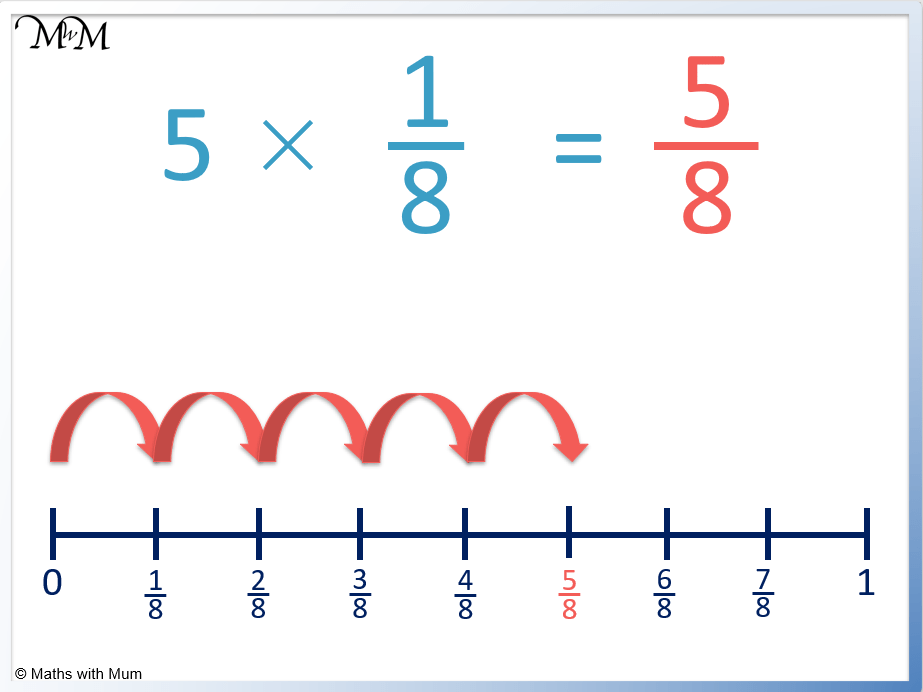5 ×   1/8   =   5/8.

Here is another example involving an improper fraction or mixed number.

Work out 5 ×   1/3   using a number line.We split each whole number into thirds. We then count five of these jumps on our number line.

5 ×   1/3   =   5/3.

As a mixed number this is 1   2/3.

## Multiplying a Fraction by a Whole Number using Models

Models can be used to teach the process of multiplying fractions by whole numbers.

Here is a model of the fraction   1/3. To multiply it by 2, we have twice as many parts.

We can see that we now have   2/3   shaded in.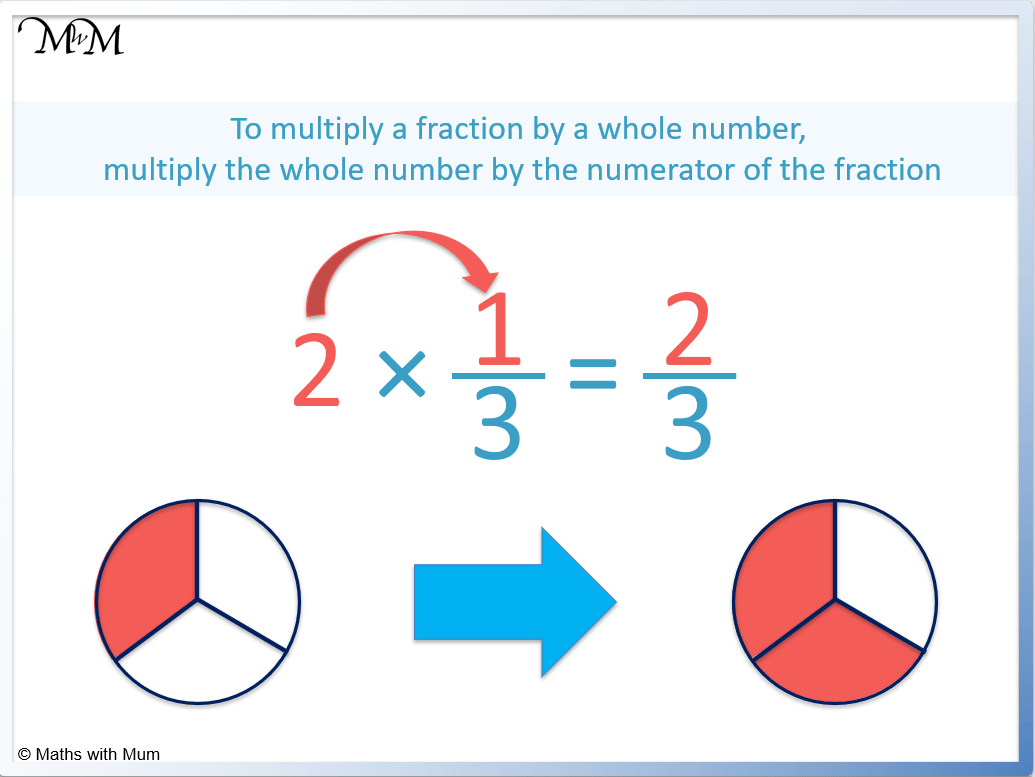Here is another example of using a model to multiply   1/4   by 3.When we multiply   1/4   by 3, we now have   3/4  .

Models are useful when multiplying fractions and whole numbers because we can see that the denominator of the fraction does not change because the number of parts in the model remains the same.

## Multiplying Fractions and Whole Numbers Word Problems

Here are some examples of word problems involving multiplying fractions and whole numbers.

Q1. I cycle   1/3   of a kilometre every day. How many kilometres do I cycle in 5 days?

Answer:   1/3   × 5 =   5/3.

Q2. At a party, each person needs   3/4   of a litre of drink. 10 people are coming in total. How many litres of drink should be bought?

Answer:   3/4   × 10 =   30/4.

Q3. My phone battery charges up by   3/100   every minute. What fraction will it have charged up after 7 minutes?

Answer:   3/100   × 7 =   21/100.Now try our lesson on Simplifying Ratios where we learn how to simplify different ratios.error: Content is protected !!